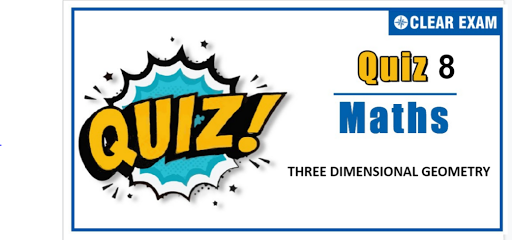## Three Dimensional Geometry Quiz

Important topics for Maths has been designed in such a way that it offers very practical and application-based learning to further make it easier for students to understand every concept or topic by correlating it with the day-to-day experiences.

Q1. A plane passing through (1, 1, 1) cuts positive direction of co-ordinate axes at A,B and C, then the volume of tetrahedron OABC satisfies
•  V≤9/2
•  V≥9/2
•  V=9/2
•  None of these
Solution
(b) Let the equation of the plane be x/a+y/b+z/c=1 ⇒1/a+1/b+1/c=1 ⇒ Volume of tetrahedron OABC=V=1/6 (abc) Now (abc)^(1/3)≥3/(1/a+1/b+1/c)≥3 (G.M.≥H.M.) ⇒abc≥27 ⇒V≥9/2

Q2.The direction cosines of a line satisfy the relations λ(l+m)=n and mn+nl+lm=0. The value of λ, for which the two lines are perpendicular to each other, is
•  1
•  2
•  1/2
•  None of these
Solution
(b) Eliminating n, we get λ(l+m)^2+lm=0 ⇒(λl^2)/m^2 +(2λ+1)l/m+λ=0 ⇒(l_1 l_2)/(m_1 m_2 )=1 (product of roots l_1/m_1 and l_2/m_2 ) Where l_1/m_1 and l_2/m_2 are the roots of this equation, further eliminating m, we get λl^2-ln-n^2=0 ⇒(l_1 l_2)/(n_1 n_2 )=-1/λ Since the lines with direction cosines (l_1,m_1,n_1) and (l_2,m_2,n_2) are perpendicular, we have l_1 l_2+m_1 m_2+n_1 n_2=0 ⇒1+1-λ=0 ⇒ λ=2

Q3.  The plane 4x+7y+4z+81=0 is rotated through a right angle about its line of intersection with the plane 5x+3y+10z=25. The equation of the plane in its new position is
•  x-4y+6z=106
•  x-8y+13z=103
•  x-4y+6z=110
•  x-8y+13z=105
Solution
(a) The equation of the plane through the line of intersection of the plane 4x+7y+4z+81=0 and 5x+3y+10z=25 is (4x+7y+4z+81)+λ(5x+3y+10z-25)=0 ⇒(4+5λ)x+(7+3λ)y+(4+10λ)z+81-25λ=0 (i) Which is perpendicular to 4x+7y+4z+81=0 ⇒4(4+5λ)+7(7+3λ)+4(4+10λ)=0 ⇒81λ+81=0 ⇒λ=-1 Hence the place is x-4y+6z=106

Q4. A line makes an angle θ with each of the x- and z-axes. If the angle β, which it makes with y-axis, is such that sin^2⁡β=3 sin^2⁡θ, then cos^2⁡θ equals
•  2/3
•  1/5
•  3/5
•  2/5
Solution
(c) Here sin^2⁡β=3 sin^2⁡θ (i) By the question, cos^2⁡θ+cos^2⁡θ+cos^2⁡β=1 (ii) ⇒ cos^2⁡β=1-2 cos^2⁡θ (iii) Adding (i) and (iii), we get 1=1+3 sin^2⁡θ-2 cos^2⁡θ ⇒1=1+3(1-cos^2⁡θ )-2 cos^2⁡θ ⇒5 cos^2⁡θ=3 ⇒cos^2⁡θ=3/5

Q6. In a three dimensional co-ordinate system, P,Q and R are images of a point A(a,b,c) in the x-y, y-z and z-x planes, respectively. If G is the centroid of triangle PQR, then area of triangle AOG is (O is the origin)
•  0
•  a^2+b^2+c^2
• 2/3(a^2+b^2+c^2)
•  None of these
Solution
(a) Point A is (a,b,c)⇒ Points P,Q,R are (a,b,-c),(-a,b,c) and (a,-b,c), respectively ⇒ Centroid of triangle PQR is (a/3,b/3,c/3)⇒G≡(a/3,b/3,c/3) ⇒A,O,G are collinear ⇒ area of triangle AOG is zero

Q7. The lines which intercept the skew lines y=mx,z=z;y=-mx,z=-c and the x-axis lie on the surface
•  cz=mxy
•  xy=cmz
•  cy=mxz
•  None of these
Solution
(c) Equating of the planes through y=mx,z=c and y=-mx,z=-c are respectively, (y-mx)+λ_1 (z-c)=0 (i) and (y+mx)+λ_2 (z+c)=0 (ii) It meets at x-axis, i.e., y=0=z ∴ λ_2=λ_1 From (i) and (ii), (y-mx)/(z-c)=(y+mx)/(z+c) ∴cy=mzx

Q8.Equation of a line in the plane π≡2x-y+z-4=0 which is perpendicular to the line l whose equation is (x-2)/1=(y-2)/(-1)=(z-3)/(-2) and which passes through the point of intersection of l and π is
•  (x-2)/1=(y-1)/5=(z-1)/(-1)
•  (x-1)/3=(y-3)/5=(z-5)/(-1)
•  (x+2)/2=(y+1)/(-1)=(z+1)/1
•  (x-2)/2=(y-1)/(-1)=(z–1)/1
Solution
(b) Let direction ratios of the line be (a,b,c), then 2a-b+c=0 and a-b-2c=0 i.e, a/3=b/5=c/(-1) Therefore, direction ratios of the line are (3,5,-1) Any point on the given line is (2+λ,2-λ,3-2λ), it lies on the given plane π if 2(2+λ)-(2-λ)+(3-2λ)=4 ⇒4+2λ-2+λ+3-2λ=4 ⇒λ=-1 Therefore, the point of intersection of the line and the plane is (1,3, 5) Therefore, equation of the required line is (x-1)/3=(y-3)/5=(z-5)/(-1)

Q9. The Cartesian equation of the plane r =(1+λ-μ) i ̂+(2-λ) j ̂+(3-2λ+2μ)k ̂ is
•  2x+y=5
•  2x-y=5
•  2x+z=5
•  2x-z=5
Solution
(c) Given plane is r =(1+λ-μ) i ̂+(2-λ) j ̂+(3-2λ+2μ)k ̂ ⇒ r=(i ̂+2j ̂+3k ̂ )+λ(i ̂-j ̂-2k ̂ )+μ(-i ̂+2k ̂) Which is a plane passing through a =i ̂+2j ̂+3k ̂ and parallel to the vectors b =i ̂-j ̂-2k ̂ and c ⃗=-i ̂+2k ̂ Therefore, it is perpendicular to the vector n =b ⃗×c =-2i ̂-k ̂ Hence, equation of plane is -2(x-1)+(0)(y-2)-(z-3)=0 or 2x+z=5

Q10. The coordinates of the point P on the line r =(i ̂+j ̂+k ̂ )+λ(-i ̂+j ̂-k ̂) which is nearest to the origin is
•  (2/3,4/3,2/3)
•  (-2/3,-4/3,2/3)
•  (2/3,4/3,-2/3)
• None of these
Solution
(a) Let the point P be (x,y,z), then the vector (xi ̂+yj ̂+zk ̂ ) will lie on the line ⇒(x-1) i ̂+(y-1) j ̂+(z-1) k ̂=-λi ̂+λj ̂-λk ̂ ⇒x=1-λ,y=1+λ and z=1-λ Now point P is nearest to the origin ⇒D=(1-λ)^2+(1+λ)^2+(1-λ)^2 ⇒dD/d=-4(1-λ)+2(1+λ)=0 ⇒ λ=1/3 ⇒ The point is (2/3,4/3,2/3)#### Written by: AUTHORNAME

AUTHORDESCRIPTION## Want to know more

Please fill in the details below:

## Latest NEET Articles\$type=three\$c=3\$author=hide\$comment=hide\$rm=hide\$date=hide\$snippet=hide

Name

ltr
item
BEST NEET COACHING CENTER | BEST IIT JEE COACHING INSTITUTE | BEST NEET & IIT JEE COACHING: Three-Dimensional-Geometry-Quiz-8
Three-Dimensional-Geometry-Quiz-8